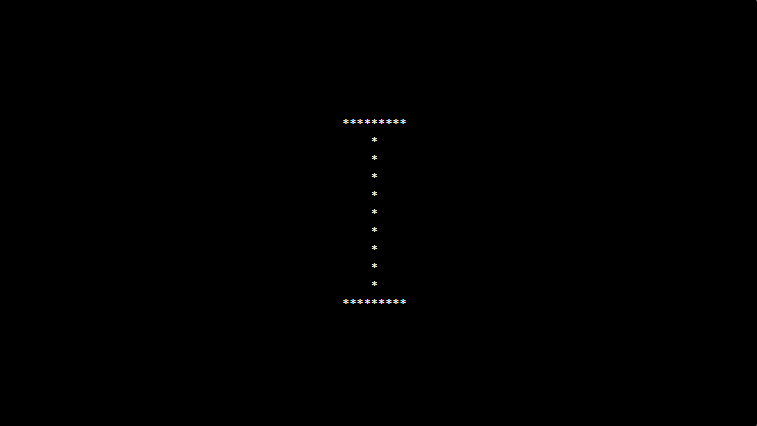# PHP program to print alphabet pattern I

In this article write a PHP program to print alphabet pattern I. This Program first takes the numbers of rows and then prints pattern using nested for loops.

## PHP program to print alphabet pattern I

```<?php
echo "<pre>";
for (\$row = 0; \$row < 11; \$row++) {
for (\$col = 0; \$col <= 11; \$col++) {
if (\$col == 5 OR (\$row == 0 AND \$col > 0 AND \$col < 10) OR (\$row == 10 AND \$col > 0 AND \$col < 10)) {
echo "*";
} else {
echo "&nbsp;";
}
}
echo "<br/>";
}
echo "</pre>";
?>
```

### Output: